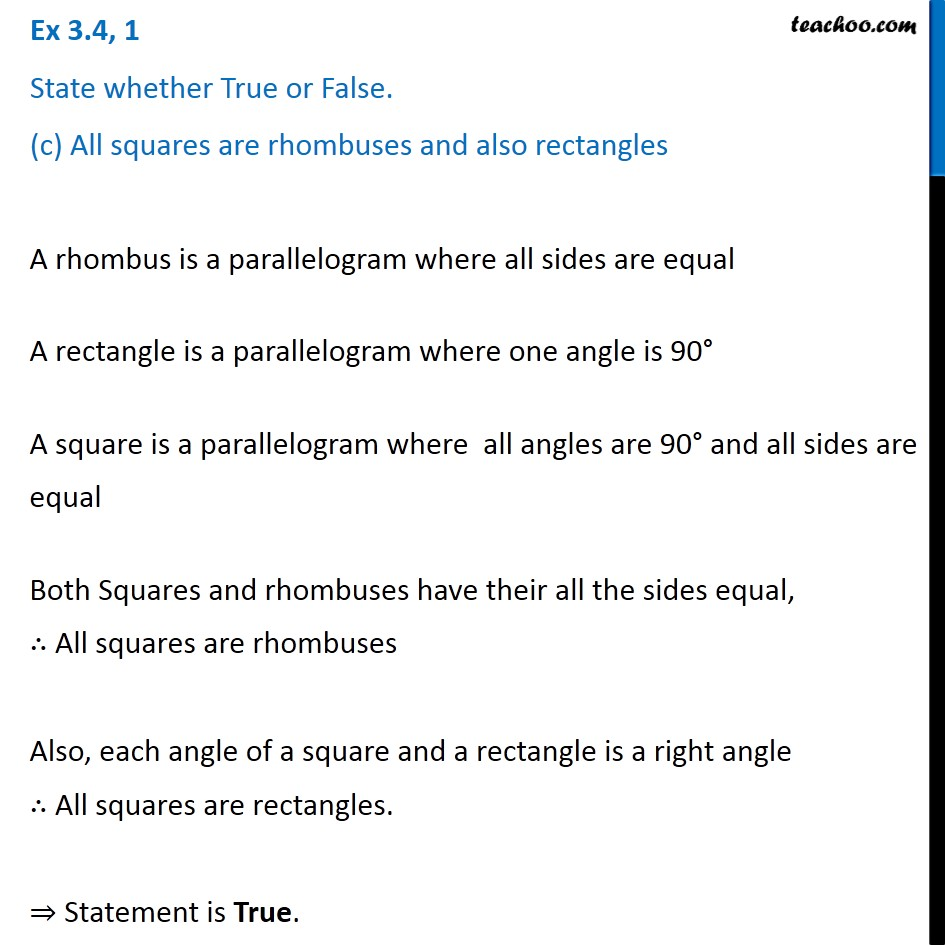Ex 3.4

Chapter 3 Class 8 Understanding Quadrilaterals
Serial order wiseLearn in your speed, with individual attention - Teachoo Maths 1-on-1 Class

### Transcript

Ex 3.4, 1 State whether True or False. (c) All squares are rhombuses and also rectangles A rhombus is a parallelogram where all sides are equal A rectangle is a parallelogram where one angle is 90° A square is a parallelogram where all angles are 90° and all sides are equal Both Squares and rhombuses have their all the sides equal, ∴ All squares are rhombuses Also, each angle of a square and a rectangle is a right angle ∴ All squares are rectangles. ⇒ Statement is True.